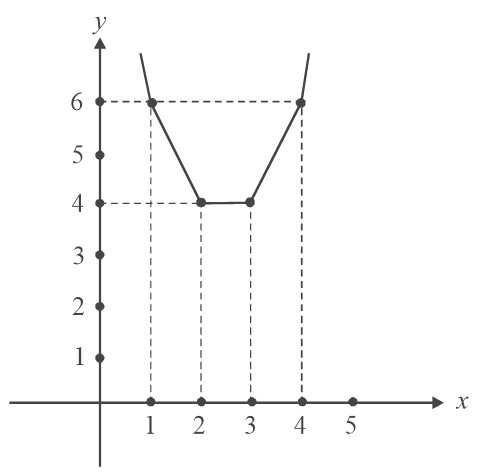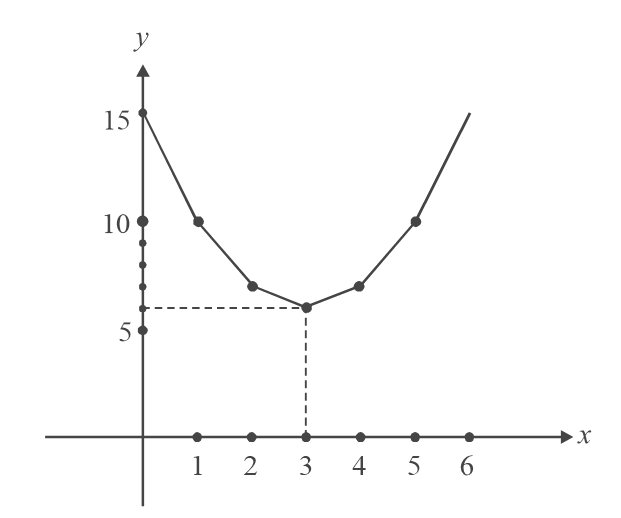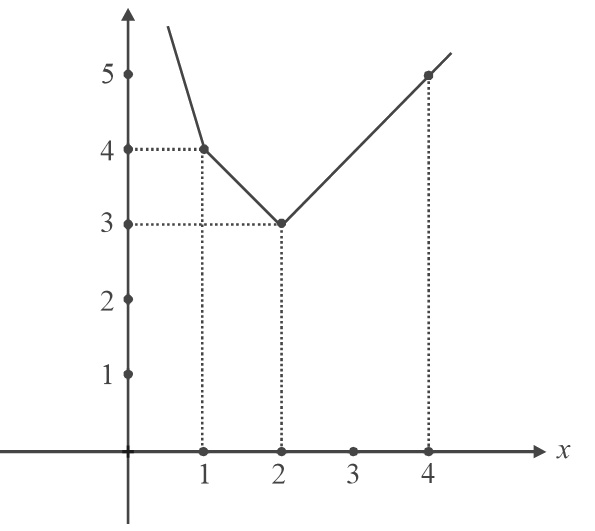# Functions Set 5

Go back to  'SOLVED EXAMPLES'

Example -8

Find the range of

 (a) y = \begin{align}{\sin ^{ - 1}}\left( {\frac{{{x^2} + 1}}{{{x^2} + 2}}} \right)\end{align} (b)  y = \begin{align}\frac{1}{{{{\sin }^4}x + {{\cos }^4}x}}\end{align} (c)   $$y = \sqrt {2 - x} + \sqrt {1 + x}$$ (d)   $$y = \sqrt {x - 1} + 2\sqrt {3 - x.}$$

Solution: (a)   Let us first evaluate the range of \begin{align}\frac{{{x^2} + 1}}{{{x^2} + 2}} = z\end{align}  (say)

z = \begin{align}\frac{{{x^2} + 1}}{{{x^2} + 2}}\,\,\, = \,\,\,\,\frac{{{x^2} + 2 - 1}}{{{x^2} + 2}}\,\,\,\, = \,\,\,\,1 - \frac{1}{{{x^2} + 2}} \ge \,\,\,1 - \frac{1}{2}\,\,\, = \,\,\,\frac{1}{2}\end{align}

(Notice the last steps carefully; \begin{align}{x^2} \ge 0\,\, \Rightarrow \,\,\,{x^2} + 2 \ge 2\end{align}   $$\Rightarrow$$ \begin{align}\frac{1}{{{x^2} + 2}} \le \frac{1}{2}\end{align}   $$\Rightarrow$$ \begin{align}- \frac{1}{{{x^2} + 2}} \ge - \frac{1}{2}\end{align}   )

Now,   $$z \ge \frac{1}{2}$$  implies \begin{align}{\sin ^{ - 1}}z \ge {\sin ^{ - 1}}\frac{1}{2} = \frac{\pi }{6}\end{align}  .

$${\sin ^{ - 1}}$$ z can take a maximum value of    \begin{align}\frac{\pi }{2}\end{align} for z = 1, but we see that for no value of x does z  become 1 (although z ‘approaches’ or ‘almost becomes’ 1 as x becomes larger and larger i.e. as $$x \to \infty ,\,z \to 1,{\text{but }}z \ne 1$$).

Hence   $${\sin ^{ - 1}}$$  1 =   $$\frac{\pi }{2}$$  is not included in the range

$R = \left[ {\frac{\pi }{6},\frac{\pi }{2}} \right)$

(b)   y =\begin{align} \frac{1}{{{{\sin }^4}x + {{\cos }^4}x}} = \frac{1}{{{{({{\sin }^2}x + {{\cos }^2}x)}^2} - 2{{\sin }^2}x{{\cos }^2}x}}\end{align}

=\begin{align} \frac{1}{{1 - 2{{\sin }^2}{{\cos }^2}x}} = \frac{1}{{1 - \frac{1}{2}{{(\sin 2x)}^2}}}\end{align}

Now      $$- 1 \le \sin 2x \le 1$$     $$\Rightarrow$$  $$0 \le {(\sin 2x)^2} \le 1$$

$$\Rightarrow$$ $$0 \le \frac{1}{2}{(\sin 2x)^2} \le \frac{1}{2}$$     $$\Rightarrow$$ $$\frac{1}{2} \le 1 - \frac{1}{2}{(\sin 2x)^2} \le 1$$

$$\Rightarrow$$  \begin{align}1 \le \frac{1}{{1 - \frac{1}{2}{{(\sin 2x)}^2}}} \le 2\end{align}

Therefore, the range is [1, 2].

(c)  y =   $$\sqrt {2 - x} + \sqrt {1 + x}$$  = $$\sqrt {2 - x} + \sqrt {x - ( - 1)}$$

This is of the form $$\sqrt {a - x} + \sqrt {x - b}$$  , which we discussed earlier.

The minimum for y comes at  $$x = {\rm{ }}-1,{\rm{ }}2$$ :      y   = $$\sqrt 3$$

The maximum comes at x = \begin{align}\frac{{ - 1 + 2}}{2}\,\,\,\,:\,\,\,\,y = \sqrt 6\end{align}

The range is R  = $$\left[ {\sqrt 3 ,\sqrt 6 } \right]$$

(d)    $$y = \sqrt {x - 1} + 2\sqrt {3 - x}$$

This function is no longer symmetric, so we carry out a standard analysis.

The domain is [1, 3]. Also, y > 0.

Now  $${y^2}$$ = $$(x - 1) + 4(3 - x) + 4\sqrt {(x - 1)(3 - x)}$$

= $$11 - 3x + 4\sqrt {(x - 1)(3 - x)}$$

Rearranging and squaring gives

$${({y^2} + 3x - 11)^2} + 16({x^2} - 4x + 3) = 0$$

$$\Rightarrow {y^4} + {(3x - 11)^2} + 2{y^2}(3x - 11) + 16({x^2} - 4x + 3)= 0$$

$$\Rightarrow 25{x^2} + (6{y^2} - 130)x + ({y^4} - 22{y^2} + 169) = 0$$

For real x, we require D > 0

$$\Rightarrow {(3{y^2} - 65)^2} - 25({y^4} - 22{y^2} + 169) \ge 0$$

$$\Rightarrow - 16{y^4} + 160{y^2} \ge 0$$

$$\Rightarrow {y^4} - 10{y^2} \le 0$$

$$\Rightarrow y \le \sqrt {10}$$

Also, at $$x = 1$$, y = $$2\sqrt 2$$   and at x = 3, y = $$\sqrt 2$$ , and therefore, the minimum value of y is $$\sqrt 2$$

The range is $$\left[ {\sqrt 2 ,\sqrt {10} } \right]$$

(we will be able to evaluate the range much more easily using derivatives later on).

Example - 09

Find the range of

$$y = \,\,|x - 1|\,\, + \,\,|x - 2|\,\, + \,\,|x - 3|\,\, + \,\,|x - 4|.$$

Solution: Let us approach this problem in two different ways.

(i)   Define it piecewise (define it separately in  each interval):

${\rm{x }} \le {\rm{1}}\qquad \quad y = 1 - x + 2 - x + 3 - x + 4 - x = 10 - 4x$

${\rm{1 }} < {\rm{x }} \le {\rm{2}}\qquad \quad y = x - 1 + 2 - x + 3 - x + 4 - x = 8 - 2x$

${\rm{2}} < x{\rm{ }} \le {\rm{3}}\qquad \quad y = x - 1 + x - 2 + 3 - x + 4 - x = 4$

${\rm{3 }} < {\rm{x }} \le {\rm{4}}\qquad \quad y = x - 1 + x - 2 + x - 3 + 4 - x = 2x - 2$

${\rm{x > 4}}\qquad\quad y = x - 1 + x - 2 + x - 3 + x - 4 = 4x - 10$

Now draw the graph:The minimum is $$y = {\rm{ }}4{\rm{ \;}}\;for\;\;{\rm{ }}2 \le x \le 3.$$ The range is $$\left[ {4,{\rm{ }}\infty } \right).$$

(ii)   Symmetry suggests that we should get a minimum for x =\begin{align} \frac{{1 + 2 + 3 + 4}}{4}\end{align}, but it is not unique. The function is minimum for the entire interval [2,3]. This is due to the nature of this function, which contains the modulus functions.

Consider the graphs of $$y = \,|x - 1|\,\, + \,\,|x - 2|\,\, + \,\,|x - 3|\,\, + \,\,|x - 4|\,\, + \,\,|x - 5|$$Here, the minimum is y = 6 at x = 3, $$\left( {\frac{{1 + 2 + 3 + 4 + 5}}{5}} \right)$$ , which is unique. Reread the above carefully to determine why the minimum is unique now (the function y = f (x) never becomes constant, as in the previous case)

Now consider the graph of $$y = \,\,|x - 1| + |x - 2| + |x - 4|$$The minimum is at x = 2, equal to y = 3 and not at  $$x = \left( {\frac{{1 + 2 + 4}}{3}} \right) = \frac{7}{3}$$ . Symmetry is wrong here!

Actually, symmetry is not wrong, but this function does not have complete symmetry (this is obvious from the graph), while the previous two functions did. This is because in functions of the form $$\sum {|x - {a_i}|,}$$  a turning point (a sharp point) will always come at one of the $${a_i}'s$$ , and the extremum will always lie at such a point. In the first function, there is a minimum at x = 2, 3 (which are sharp points) and hence at all the points in between. In the second function, there is a minimum at x = 3 (a sharp point) which happens to be the same as $$\frac{{\sum {{a_i}} }}{n}$$ (because the points 1, 2, 3, 4, 5 are symmetrically placed about x = 3).

In the third function, the minimum is at x = 2 but $$\sum {{a_i}} /n$$ lies somewhere else, because the points 1, 2, 4 are not distributed symmetrically about any of these sharp points

So for example   $$f = \,|x + 1| + |x| + |x - 1|$$  has 3 sharp point, {–1, 0, 1} distributed symmetrically about one of the sharp point {0}, and hence the minimum is at x = 0 equal to y = 2, which is also obtained by symmetry.

$$f = |x + 1| + |x| + |x - 2|$$ has 3 sharp points {–1, 0, 2} which are not distributed symmetrically about any sharp point and hence, although   \begin{align}\frac{{ - 1 + 0 + 2}}{3} = \frac{1}{3}\end{align} , the minimum is at 0 and not 1/3.

$$y = |x - 2| + |x - 1| + |x + 1| + |x + 2|$$ has 4 sharp points {–2, –1, 1, 2} which are distributed symmetrically about –1 and 1. The minimum is therefore at \begin{align}\frac{{ - 2 + - 1 + 1 + 2}}{4} = 0\end{align}  (and also at all values between –1 and 1 since the function assumes a constant value in this interval (y = 6).)

Therefore, we see that symmetry has to be used carefully.

-  For example, a function of the form $$\sum {|x - {a_i}|,}$$ could be non-symmetric but a minimum could still occur at $$\sum {{a_i}} /n$$ , when the number of   $${a_i}'s$$ are even. You need not get confused with all these arguments above. Just remember to use symmetry with caution!)

-  One could argue that the minimum for    $${(x - {a_1})^2} + {(x - {a_2})^2} + {(x - {a_n})^2}$$ should come at one of the points  $${a_1},{a_2}.......{a_n}$$ and not at \begin{align}\frac{{{a_1} + {a_2} + ..... + {a_n}}}{n}\end{align}  as we saw earlier. But this is false since this function does not have sharp points’. It is hence not necessary that the extremum will be at one of the points $${a_1},{a_2}.......{a_n}$$  . As we will see later on, this function is differentiable, meaning that it is smooth, while $$\sum {|x - {a_i}|}$$   is non differentiable, meaning it is not smooth).

Learn from the best math teachers and top your exams

• Live one on one classroom and doubt clearing
• Practice worksheets in and after class for conceptual clarity
• Personalized curriculum to keep up with school